# FFT analyser

When you press the Setup button on FFT analyser, the following setup window will open: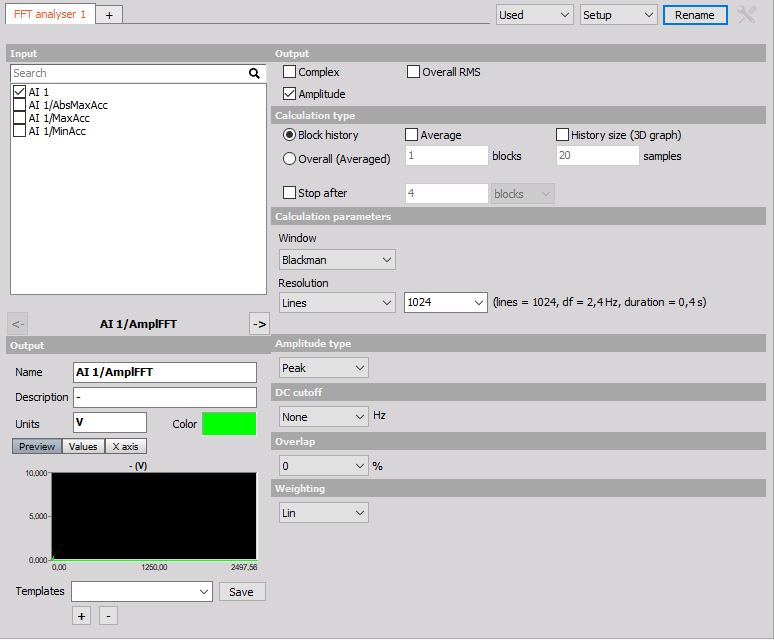## Output

• Complex - outputs are phase, imaginary and real part of the signal.
• Overall RMS - output is only one value for the whole measurement.
• Amplitude - output is the amplitude of the signal.

## Calculation type

• Block history - uses blocks to calculate FFT spectrum.
• Overall (Averaged) - gives only one averaged FFT spectrum at the end of the measurement. It will average all the blocks in the signal and the output will be only one FFT for the whole measurement.

## Calculation parameters

• Window - Rectangular, Hanning, Hamming, Flat Top, Triangle, Blackman, Exponent down, Transient and Backman-Harris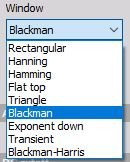• Resolution - defined in the number of Lines, with the delta frequency Df [Hz] or with Block duration [s].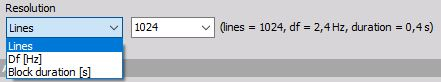## Amplitude type

The Amplitude type display section defines display in Y-amplitude axis.

In Amplitude type we can choose from:

• Amplitude - is the pure signal amplitude [V].
• RMS - is the RMS amplitude, calculated as Amplitude/sqrt(2) [V].
• Power - calculated as RMS value squared [V*V]. PSD - calculated as RMS squared, divided by the line resolution and sqrt(2), used for checking the noise [V*V/Hz]. RMS SD - calculated as RMS value, divided by the square root of line resolution - also used for checking the noise [V/sqrt(Hz)].

## DC cutoff

To remove DC or low-frequency components, select from a drop-down list the DC cutoff filter - lower limit.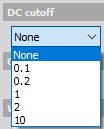## Overlap

Overlap defines the percentage of time signal that has already been calculated and it is used again for calculation (example: 50% overlapping means that the calculation will take half of the old data).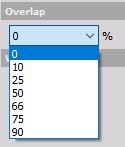## Weighting

As a standard, FFT analyser uses Linear Weighting. For sound analysis, special FFT weighting can be set. As opposed to the sound module in math, where the weightings will be calculated in the time domain, this will calculate the sound weighting in the frequency domain. Weighting can be of the following type:

• Linear weighting - is linear at all frequencies and it has the same effect on all measured values.
• A weighting - is applied to instrument-measured sound levels in an effort to account for the relative loudness perceived by the human ear, as the ear is less sensitive to low audio frequencies.
• B weighting - is used for intermediate levels and is similar to A, except for the fact that low-frequency attenuation is a lot less extreme though still significant (-10 dB at 60 Hz). This is the best weighting to use for music listening purposes.
• C weighting - is similar to A and B as far as the high frequencies are concerned. In the low-frequency range, it hardly provides attenuation. This weighting is used for high-level noise.
• D weighting - was specifically designed for use when measuring high-level aircraft noise. The large peak in the D-weighting curve is not a feature of the equal-loudness contours but reflects the fact that humans hear random noise differently from pure tones, an effect that is particularly pronounced around 6 kHz.

## FFT markers

2D graph can display values of the currently selected point with the crosshair cursor. When clicking on such point with the left mouse button, the marker line will be added showing x axis value on the x-axis and showing y-axis vale of a certain point above the marked point. All points can be removed by pressing the right mouse button and select Delete selected marker.

For detailed information about FFT markers see -> 2D Graph.

For additional help visit Dewesoft PRO training  -> Dewesoft Web page -> PRO Training -> FFT markers.

For detailed information about FFT Analysis see -> FFT Analysis.